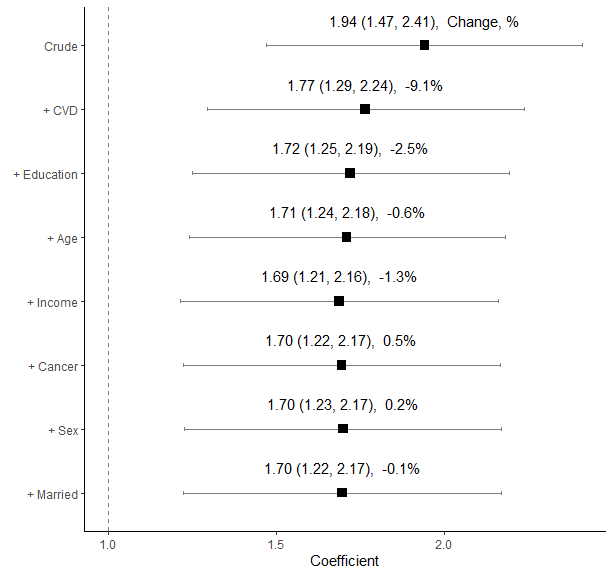# Change-in-estimate Approach: Assessing Confounding Effects

Badges Confounding; Logistic regression; Cox proportional hazards model; Linear regression;

## Overview

The ‘chest’ package systematically calculates and compares effect estimates from various models with different combinations of variables. It calculates the changes in effect estimates when each variable is added to the model sequentially in a step-wise fashion. Effect estimates here can be regression coefficients, odds ratios and hazard ratios depending on modelling methods. At each step, only one variable that causes the largest change among the remaining variables is added to the model. The final results from many models are summarized in one graph and one data frame table. This approach can be used for assessing confounding effects in epidemiological studies and bio-medical research including clinical trials.

## Installation

You can install the released version of chest from CRAN with:

install.packages("chest")

## Getting Started

library(chest)
library(ggplot2)
names(diab_df)
#>   "Endpoint"  "mid"       "Diabetes"  "Age"       "Sex"       "BMI"
#>   "Married"   "Smoke"     "CVD"       "Cancer"    "Education" "Income"
#>  "t0"        "t1"

### Data: diabetes and mortality

A data frame ‘diab_df’ is used to examine the association between Diabetes and mortality Endpoint. The purpose of using this data set is to demonstrate the use of the functions in this package rather than answering any research questions.

### ‘chest_speedglm’: report odds ratios at all steps with logistic regression models

results <- chest_speedglm(
crude = "Endpoint ~ Diabetes",
xlist = c("Age", "Sex", "Married", "Smoke", "Cancer", "CVD","Education", "Income"),
data = diab_df)
results$data #> variables est lb ub Change p n #> 1 Crude 2.305786 1.809862 2.937600 NA NA 2048 #> 2 + Age 3.297099 2.421755 4.488837 42.99238627 NA 2048 #> 3 + Income 2.902046 2.125534 3.962238 -11.98183743 NA 2048 #> 4 + CVD 2.797882 2.044220 3.829405 -3.58931015 NA 2048 #> 5 + Smoke 2.900600 2.113218 3.981361 3.67127605 NA 2048 #> 6 + Sex 2.872333 2.092484 3.942824 -0.97453037 NA 2048 #> 7 + Married 2.894543 2.107594 3.975330 0.77324421 NA 2048 #> 8 + Cancer 2.881019 2.097209 3.957769 -0.46723505 NA 2048 #> 9 + Education 2.878757 2.093150 3.959219 -0.07851916 NA 2048 We can also use ‘chest_plot’ to show the results above as a ‘ggplot’ object. results <- chest_speedglm( crude = "Endpoint ~ Diabetes", xlist = c("Age", "Sex", "Married", "Smoke", "Cancer", "CVD","Education", "Income"), data = diab_df) chest_plot(results)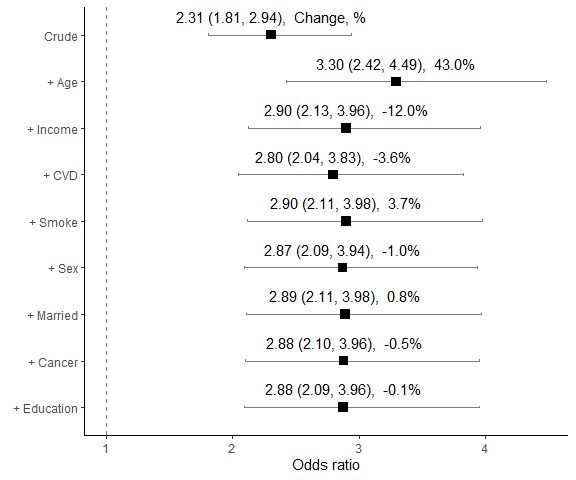We adjust the location of the values. results <- chest_speedglm( crude = "Endpoint ~ Diabetes", xlist = c("Age", "Sex", "Married", "Smoke", "Cancer", "CVD","Education", "Income"), data = diab_df) p <- chest_plot(results, nudge_y = 0, value_position = 5) p + scale_x_continuous(breaks = c(0.5, 1:4), limits = c(0.5, 8))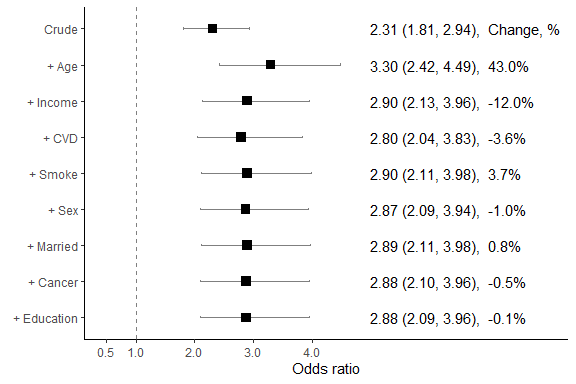Or remove the values. results <- chest_speedglm( crude = "Endpoint ~ Diabetes", xlist = c("Age", "Sex", "Married", "Smoke", "Cancer", "CVD","Education", "Income"), data = diab_df) chest_plot(results, no_values = TRUE)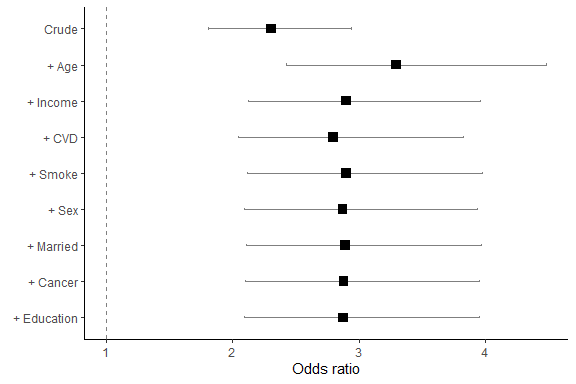Users can also use ‘chest_forest’ to call ‘forestplot’ package. results <- chest_speedglm( crude = "Endpoint ~ Diabetes", xlist = c("Age", "Sex", "Married", "Smoke", "Cancer", "CVD","Education", "Income"), data = diab_df) chest_forest(results)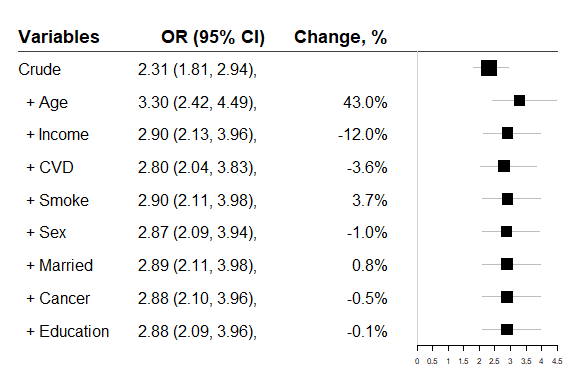All Odds ratios are for the association between Diabetes and mortality Endpoint after each of other factors added to the model sequentially. • Step 1: Started with a model: speedglm(endpoint ~ diabetes). The odds ratio for diabetes was presented in the row marked as Crude. • Step 2: Each of 8 variables was separately added to the above model, and chest_speedglm compared odds ratios from those eight models to identify the one which created the largest change. The variable Age was selected and added to the model. • Step 3: Repeat Step 2 with the remaining 7 variables. The variable income was selected and added. • Step 4 to Step 9 repeated the same procedure until all variables were added. We can see after adding age and income variables add other variables had little impact on the odds ratio estimates, and odds ratio estimates remained positive on the right hand side of non-effect line. In this case, ‘chest’ shows one table with the results after fitting 37 total models: 1 crude model plus 36 (8 + 7 + 6 +5 + 4 + 3 + 2 + 1) models. ### When the list of variables is long, or the same list to be used repeatedly, generate a object of variable list: vlist <- c("Age", "Sex", "Married", "Smoke", "Cancer", "CVD","Education", "Income") results <- chest_speedglm( crude = "Endpoint ~ Diabetes", xlist = vlist, data = diab_df) results$data
#>       variables      est       lb       ub       Change  p    n
#> 1         Crude 2.305786 1.809862 2.937600           NA NA 2048
#> 2         + Age 3.297099 2.421755 4.488837  42.99238627 NA 2048
#> 3      + Income 2.902046 2.125534 3.962238 -11.98183743 NA 2048
#> 4         + CVD 2.797882 2.044220 3.829405  -3.58931015 NA 2048
#> 5       + Smoke 2.900600 2.113218 3.981361   3.67127605 NA 2048
#> 6         + Sex 2.872333 2.092484 3.942824  -0.97453037 NA 2048
#> 7     + Married 2.894543 2.107594 3.975330   0.77324421 NA 2048
#> 8      + Cancer 2.881019 2.097209 3.957769  -0.46723505 NA 2048
#> 9   + Education 2.878757 2.093150 3.959219  -0.07851916 NA 2048
chest_plot(results)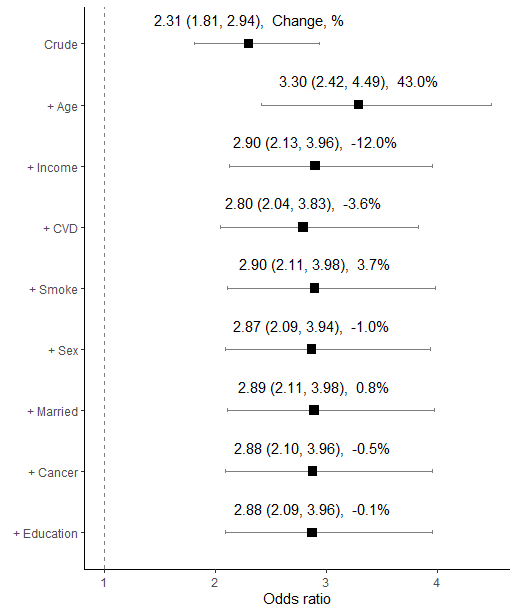## Add terms such as an interaction between Age and Sex, and age squared

diab_df$Age_Sex <- diab_df$Age*diab_df$Sex diab_df$Age2 = diab_df\$Age^2
vlist_1<-c("Age", "Sex", "Age2", "Age_Sex", "Married", "Cancer", "CVD", "Education", "Income")
results <- chest_speedglm(crude = "Endpoint ~ Diabetes", xlist = vlist_1, data = diab_df)

chest_plot(results)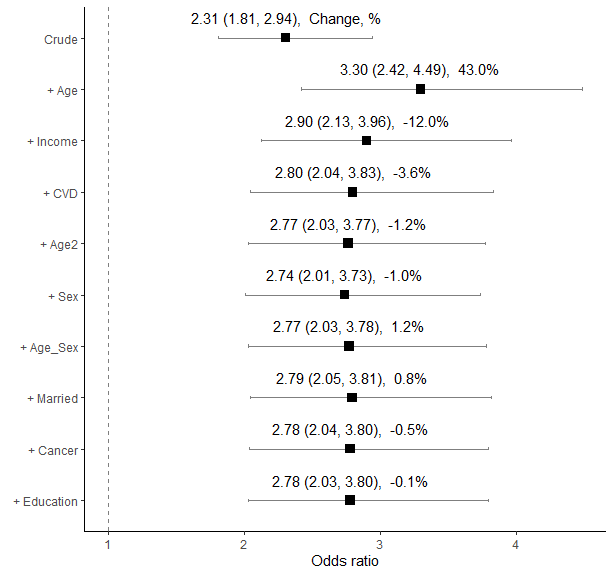chest_forest(results)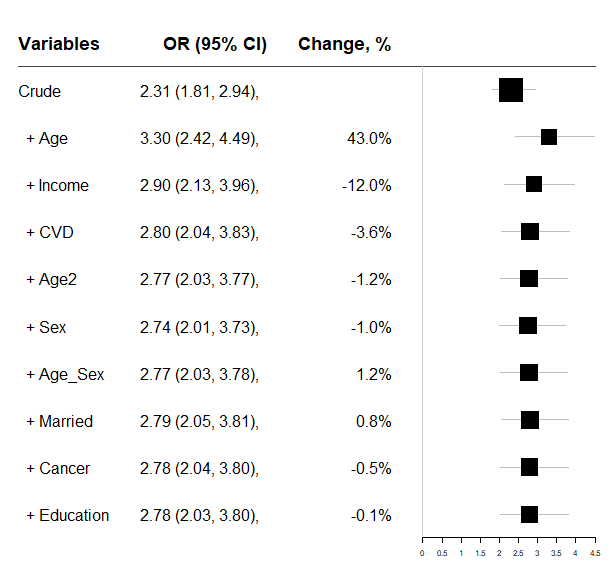### chest_glm: Logistic regression using (generalized linear models, glm).

‘chest_glm’ is slower than ‘chest_speedglm’. We can use indicate = TRUE to monitor the progress. If it is too slow, you may want to try ‘chest_speedglm’.

vlist <- c("Age", "Sex", "Married", "Smoke", "Education")
results <- chest_glm(crude = "Endpoint ~ Diabetes", xlist = vlist,
data = diab_df, indicate = TRUE)

### chest_cox: Using Cox Proportional Hazards Models: ‘coxph’ of ‘survival’ package


results <- chest_cox(crude = "Surv(t0, t1, Endpoint) ~ Diabetes",
xlist = vlist, data = diab_df)
chest_plot(results)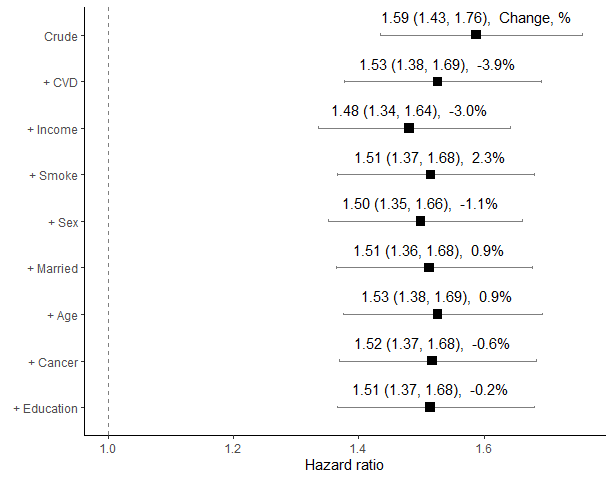chest_forest(results)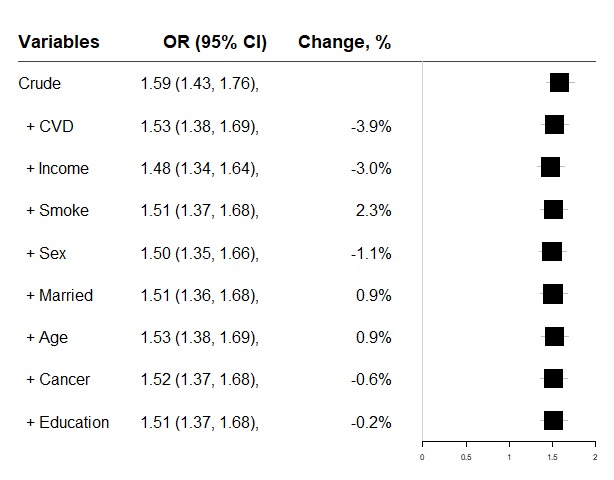### chest_clogit: Conditional logistic regression: ‘clogit’ of ‘survival’ package

results <- chest_clogit(crude = "Endpoint ~ Diabetes + strata(mid)",
xlist = vlist, data = diab_df)

### chest_lm: linear regression

vlist<-c("Age", "Sex", "Married", "Cancer", "CVD","Education", "Income")
results <- chest_lm(crude = "BMI ~ Diabetes", xlist = vlist, data = diab_df)
chest_plot(results)